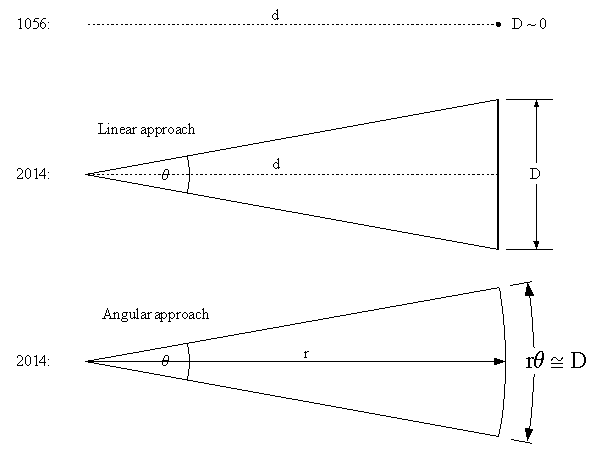# Distance to Crab Nebula.

Daniel Luo

## Homework Statement

Hi.

The Crab Nebula which can be seen with even a small telescope, is the result of a supernova. All parts of the nebula are moving away from the centre at 1.18*10^6 m/s. The angle that the Crab Nebula forms with the Earth is 0.083 degrees. This supernova was observed by chinese astronomers in 1054. You can assume that the Crab Nebula has expanded at a uniform rate since then.

- What is the distance from Earth to Crab Nebula?

## Homework Equations

Distance = Speed of light * Time elapsed.

## The Attempt at a Solution

I said that there was 2014-1054=960 years since we first observed it. Hence 960 lightyears which is equal to 9.1*10^18 m. However, this is wrong according to the answers (and wikipedia while we're at it). Btw, what do I need the expansion speed for? It's so small compared to c. And what about the angle? Any help is appreciated. Thank you.

Basic_Physics
Use a bit of trig to figure this out. It always helps to draw a diagram if the problem lends itself to it. Fill the known quantities in on the diagram. Upload it if you are still having problems.

Daniel Luo
I did that already... And I get the same answer... What am I doing wrong?

Basic_Physics
Draw the triangle that the Crab Nebula makes with the earth. Fill in the angle and known distance and upload the image if you can.

Daniel Luo
I don't think it will be much help to you. It's reversed.

Please can't you just tell me what I'm doing wrong?

#### Attachments

•Photo on 15-10-13 at 15.26.jpg
56.7 KB · Views: 434
Basic_Physics
You can get the diameter, D, of the CN since it has expanding for 960 years using its expansion rate. Together with the angle that D extends at the earth you can then calculate R, the distance to the CN. Hint: Work in radians.

Daniel Luo
I simply do not understand what you just wrote. The diameter is 2 * 1.18*10^6 m/s * 960 years * 31557600 years/s = 7.15 * 10^16 m. What do I do from here?

Basic_Physics
For an angular measurement, θ, in radians, we have that

θ = s/r

where s is the arc length, D in this case, and r is the radius of the circle, the distance to the CN in this case.

Daniel Luo
How can the radius be the distance from CN to Earth? Isn't the radius simply the radius of crab nebula? And how is the arc length how much CN has expanded the last 960 years?

Basic_Physics
The given angle is the angle that the diameter of the nebula subtends at the earth, as you indicated on your diagram. So if we work in radians then the r in the formula above is the distance to the nebula and the arc length, s in the formula, is the diameter of the nebula.

Daniel Luo
Couldn't you also say that the radius is the opposite side in the right angled triangle. The adjacent side is the distance from CN to Earth, and the 0.083/2=0.0415 degrees is the angle. Since tan(0.0415) = radius/distance we have that the distance is radius/tan(0.0415). This gives the right answer.

Mentor
The methods are equivalent. The angular approach is more common in astronomy where almost everything is measured in angular terms.#### Attachments

•1 person